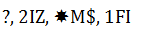## Alpha-Numeric Series Questions For SBI PO/Clerk Prelims

Alpha-Numeric Series Questions For SBI PO/Clerk Prelims. Welcome to the www.letsstudytogether.co online Reasoning section. If you are preparing for SBI PO/Clerk, IBPS PO/Clerk, NABARD, and IBPS exams, you will come across a section on Reasoning Ability Section. Here we are providing you with Reasoning quiz “Alphanumeric Series Questions for SBI PO/Clerk Prelims” based on the latest pattern for your daily practice.

This “Alphanumeric Series Questions for SBI PO/Clerk Prelims“ is also important for other banking exams such as SBI PO, IBPS Clerk, SBI Clerk, IBPS RRB Officer, IBPS RRB Office Assistant, IBPS SO, SBI SO and other competitive exams.

## Alpha-Numeric Series Questions for SBI PO/Clerk Prelims: Set-10

Directions:(1-5)Study the following arrangement carefully and answer the questions given below:1.Which of the following is sixth to the left of the fifteenth from the left end of the given arrangement?

A. 2
B. #
C. %
D. \$
E. None of these

C. %2. How many such numbers are there in the given arrangement each of which is immediately preceded by a consonant but not immediately followed by a letter?
A. One
B. None
C. Five
D. Two
E. Four

A. One3. How many such symbols are there in the arrangement, each of which is immediately followed by a letter but not immediately preceded by a number?
A. One
B. Three
C. None
D. Two
E. More than three

B. Three4. What should come in place of question mark (?) in the following series based on the above arrangement?

IM3     @U5    WYB    ?
A. 69H
B. #D8
C. 6#9
D. #9D
E. None of these

B. #D85. Four of the following five are alike in a certain way based on their positions in the given arrangement and so from a group. Which is the one that does not belong to that group?

A. BE6
C. 9DH
D. 5V1
E. #ZG

E. #ZG

Directions:(6-10) Answer the following questions referring to the symbol-letter-number sequence given below:6. Which of the following is exactly midway between the tenth element from the right and fifth element from the left end?
A. I
B. Z
C. 1
D. *
E. Either (a) or (b)

E. Either (a) or (b)

7. How many letters are there in the above sequence which are immediately preceded by a number and immediately followed by a consonant?
A. None
B. One
C. Two
D. Three
E. None of these

C. Two

5KL ,3GF

8.What should come in place of the question mark (?) in the following sequence?A. K\$F
B. KNF
C. LN5
D. 5\$M
E. None of these

A. K\$F

5\$D

9. Which of the following is the eleventh element to the right of the second element from the left end in the above sequence?
A. 9
B. 1
C. M
D. 4
E. None of these

10. If both the halves of the above sequence are written in reverse order, which will be the sixth element to the right of the sixteenth element from the right end?
A. 4
B. ~
C. O
D. N
E. None of these